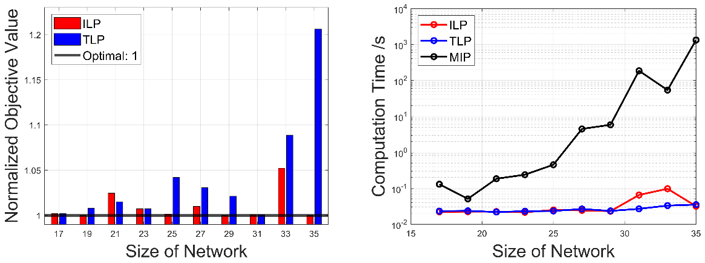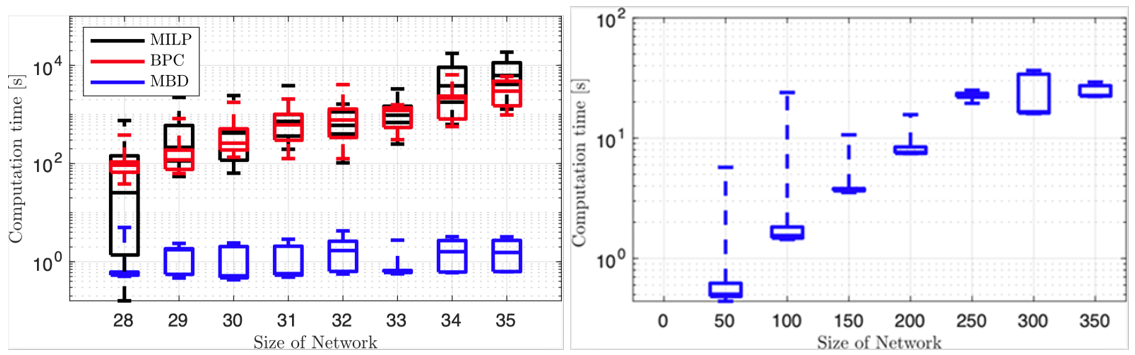Researchers achieve new advances in intelligent transportation system
Canqi YAO | 06/23/2022

Nowadays, the transportation and logistics industry has adopted numerous electric vehicles (EVs) as means of transportation. However, unlike traditional fuel vehicles, it takes a long time to charge electric vehicles, which inevitably affects the scheduling of EVs. Thus, the EV routing problem (EVRP) that jointly optimizes the charging and routing process of EVs is becoming increasingly popular, which should be solved in an online fashion for practical requirements.Aiming to solve this complicating EVRP efficiently, Professor Zaiyue Yang’s group from the Southern University of Science and Technology (SUSTech), modeled the EVRP as a mixed integer programming (MIP) problem.

Since the MIP problem is an NP-hard problem, to reduce the computational burden of solving such a MIP problem, Prof. Yang’s group proposed a two-stage algorithm (TLP) with low computational time complexity, which decomposes the original MIP problem into two linear programming problems. With the proposed TLP, an approximate optimal solution can be obtained within the polynomial solution time. In addition, to further improve the quality of the solution, an iterative algorithm (ILP) based on the TLP was proposed. In Figure 1 (left side), it shows the performance of ILP, where the maximum optimality gap is only 5%. In Figure 1 (right side), both TLP and ILP reduce the computation time by 4 orders of magnitude.Figure 1. The objective value (left) and computation time (right)

To address the uncertainty coming from future information in online EVRP, the researchers proposed a rolling horizon method and the strategy of virtual depots. In Figure 2 (left side), compared with commercial solvers such as CPLEX, GUROBI, and branch and price algorithm, the proposed MBD algorithm reduces the computation time by 3 or 4 orders of magnitude, and takes less memory for the distributed implementation. In Figure 2 (right side), the proposed algorithm can solve the instance with up to a maximum of 350 nodes and 35 vehicles, which demonstrates the superiority of the proposed algorithm.Figure 2. The computation time of different algorithms (left) and computation time over large-scale instances (right)

These two papers have been published in IEEE Transactions on Intelligent Transportation Systems (T-ITS), a top journal about the design, analysis, and control of information technology as it is applied to transportation systems.

Canqi Yao, a Ph.D. student of SUSTech and HIT, is the first author of this paper. Prof. Zaiyue Yang and Research Asst. Prof. Shibo Chen are the corresponding authors. SUSTech is the first affiliation of this paper.

This research was funded by the Key R&D Program of the Ministry of Science and Technology, the National Natural Science Foundation of China (NSFC), and the Shenzhen Science and Technology Innovation Commission.

Paper links (In order of appearance above):

2022, 06-23
By Canqi YAO